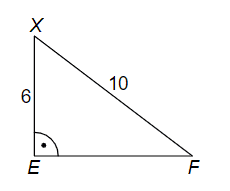# Triangle and hyperbola

charlie05

## Homework Statement

Points E and F are the focuses of the hyperbola and point X are on the hyperbola. Determine the size of the main and minor half-axes of the hyperbola.x2 = e2 - f2
x = 8

## The Attempt at a Solution

I think that eccentricity is 4 units (x/2). But I don’t know how to continue.

#### Attachments

Homework Helper
How is the hyperbola defined? What is the equation of the hyperbola?

charlie05
A hyperbola is a set of points, such that for any pointof the set, the absolute difference of the distances to two fixed points F1, F2 is constant = 2a

equation...f(x)=1/x

Homework Helper
A hyperbola is a set of points, such that for any pointof the set, the absolute difference of the distances to two fixed points F1, F2 is constant =
Correct, What is the name for a?
What is the value of a of the hyperbola shown in the problem?
equation...f(x)=1/x
That is one special hyperbola. Is it the same as the one in the problem?

charlie05
a is semi major axis
use PF2-PF1=2a?
XF-EX=2a...10-6=2a...a=2cm ?

Homework Helper
a is semi major axis
use PF2-PF1=2a?
XF-EX=2a...10-6=2a...a=2cm ?
Correct!
Where do the semimajor and minor axes appear in the equation of the hyperbola?
Can you draw a hyperbola, with axes parallel with the coordinate axes? What is the equation of such hyperbola?

charlie05
[x^2/a^2] - [y^1/b^2] = 1

a - semimajor axe
b - minor axe

Last edited:
Homework Helper
[x^2/a^2] - [y^1/b^2] = 1

a - semimajor axe
b - minor axe
It should be x^2/a^2 - y^2/b^2 = 1
You know a, and the coordinates of the point X : Substitute into the equation of the hyperbola. Calculate b.